# Sabathé mixed cycle

The actual operating conditions of diesel engines differ markedly from those that are represented in the ideal Otto and Diesel cycles. For diesel engines, the combustion process transformational approaches a constant pressure only in case of unusually large motors and slow.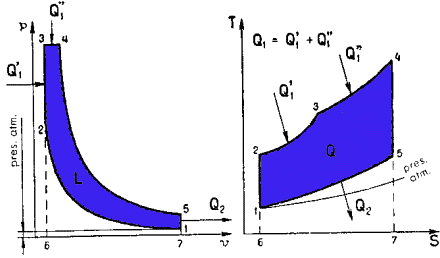The actual plot shows that, under normal conditions, the combustion takes place, in Diesel engines, according to a process that approximates a transformational combustion and a constant volume at pressures n constant.

We can say that, in practice, Otto and Diesel cycles are very close in form, to the extent they can be considered as a particular case of combined cycle, in which part of the combustion is verified at constant volume and partly at constant pressure. This theoretical cycle is represented in Figure 28 and is known Sabathé mixed cycle.

In this cycle, after the adiabatic compression stage 1-2 occurs, as in the Otto cycle, a phase of constant volume combusted 2-3, during which he enters the amount of heat Q1 'and then, as in the diesel cycle, a phase of 3-4 combusted constant pressure, is introduced in the course of which the amount of heat Q1''.

There follow two successive stages, namely a, 4-5 adiabatic expansion, and another subtraction constant volume 5.1, the amount of heat Q2.

Therefore, the total amount of heat introduced better

Q1 = Q1 '+ Q1''

Recalling the above, regarding Otto and Diesel cycles, we can write:

Q1 '= Cv (T3-T2)

Q1'' = Cp (T4-T3)

Q2 = Cv (T5-T1)

Thus, the ideal thermal performance Sabathé theoretical cycle goes:

I = (heat supplied - heat subtracted) / heat supplied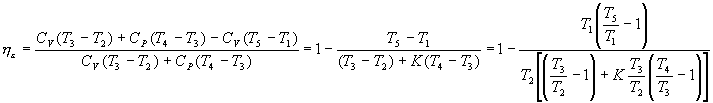For transformation combusted 2-3 constant volume we have: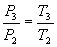Y for processing constant pressure combusted 3-4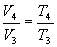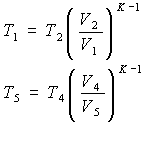For transformations 1-2 adiabatic expansion compresióny employ 4-5, respectively, formulas

Which we obtain: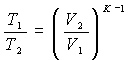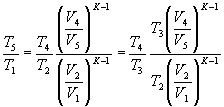And being V3 = V2, V5 = V1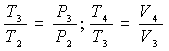You can write: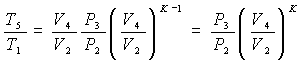Substituting these expressions in ideal thermal performance, it:Start with the relationship between the pressure P3 at the end and the pressure P2 at first combusts phase-constant volume which we will call "constant volume ratio combusted" -, and recalling that: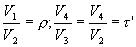We obtain the final expression of ideal thermal performance of the theoretical cycle Sabathé: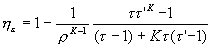A compresiónr equal ratio, the performance of the combined cycle is intermediate between that of the Otto cycle and the Diesel cycle. Increasing the heat supplied at a constant volume, that is, between points 2 and 3 and is reduced at constant pressure supplied between points 3 and 4, the thermal performance approaches that of the Otto cycle. If, however, reduces the heat supplied at constant volume and increases the corresponding constant pressure combined cycle performance approaches that of the Diesel cycle.

valoración: 3.2 - votos 20

Last review: February 1, 2016